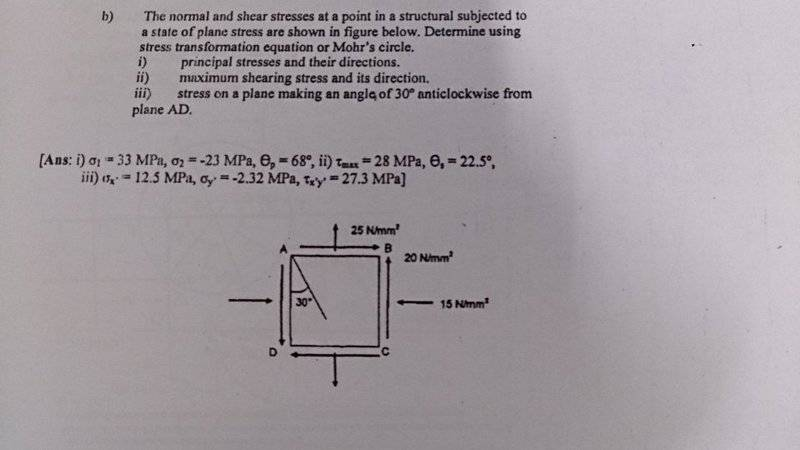# Mohr's circle

fonseh

## Homework StatementWhy cant i use the equation to determine the normal stress and shear stress ? Is my concept wrong ? I use the method in the link to do this question. The question said the
stress on a plane making angle 30 degree anticlockwise from plane AD .

## The Attempt at a Solution

When it's anticlockwise , the angle is positive
http://www.dummies.com/education/science/using-mohrs-circle-to-find-principal-stresses-and-angles/
Mod note: Image of work has been deleted. OP will be including work in a subsequent post.[/B]

Last edited by a moderator:

## Answers and Replies

fonseh
$$\sigma _(max)$$ = ((25-15 )/2 ) + 20cos60 + 20sin60 = 32.3MPa

$$\sigma x_(min)$$ = ((25-15 )/2 ) - 20cos60 - 20sin60 = -22.3MPa

But the ans is 12.5 and -2.3MPa

fonseh
##r = sqrt ( ((\sigma _x -s \sigma_y) /2 )^2)+ (\tau_xy)^2) = 28##

##tan2\theta_p = 2\tau_xy / (\sigma _x -s \sigma_y)
= 2(20) / 40## , ##\theta_p = 22.5## degree ,
This is different from the ##\theta_p ## = -68 degree given

Last edited by a moderator:
fonseh
Is the ans provided wrong ? Or is my concept wrong ?

fonseh
bump